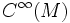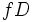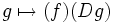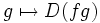# Sheaf of differential operators

This article defines a basic construct that makes sense on any differential manifold
View a complete list of basic constructs on differential manifolds

## Definition

The sheaf of differential operators of a differential manifold can be defined in many ways:

• It is the sheaf-theoretic algebra of differential operators for the sheaf of differentiable functions (in other words, if one views the sheaf of differentiable functions abstractly as a sheaf of$\R$-algebras, then the sheaf of differential operators is the sheaf-theoretic analogues of its algebra of differential operators)
• It associates to every open set, the algebra of differential operators of the$\R$-algebra of differentiable functions on that open set. Restriction maps are defined in the usual fashion.
• It is the sheaf generated, on every open set, by the algebra of differentiable functions, and the derivations

## Construction and structure

### Construction starting from derivations and multiplications

For simplicity, we restrict to the case where the open subset is the whole manifold$M$. A similar argument works for every open subset of the manifold.

We start with the algebra of infinitely differentiable functions of the manifold$M$. This is a commutative$\R$-algebra, and is denoted as$C^\infty(M)$.

We now look at the set of all derivations from$C^\infty(M)$ to itself. This is not an algebra, since the composite of two derivations need not be a derivation. It, however, turns out to be a Lie algebra, and is precisely the Lie algebra of vector fields on$M$. This is a left$C^\infty(M)$-module: post-composing a derivation with a multiplication gives a derivation. In other words:

If$f$ is a function and$D$ is a derivation, then$fD$, defined as:$g \mapsto (f)(Dg)$

is also a derivation.

However, composing on the right with a function multiplication does not yield a derivation:$g \mapsto D(fg)$

is not a derivation.

Instead of looking at the derivations alone, it makes sense to look at the$C^\infty(M)$-module comprising those operators that can be obtained as the sum of a derivation and a multiplication. In other words, maps of the form:$g \mapsto Dg + fg$

It turns out that this is a$C^\infty(M)$-bimodule, and is termed the module of first-order differential operators (the corresponding sheaf is termed the sheaf of first-order differential operators).

The first-order differential operators are not closed under composition. In other words, a composite of first-order differential operators is not necessarily a first-order differential operator. The algebra generated by first-order differential operators under composition, is precisely the algebra of differential operators.

### Natural filtration

The algebra of differential operators on$M$ has a natural filtration, in terms of the order of a differential operator. A differential operators is said to have order$\le r$ if it can be expressed as a sum of differential operators, each of which is a composite of at most$r$ first-order differential operators.

There is no natural gradation to the algebra of differential operators, because there is no way to separate a pure order$r$ component of a differential operator.